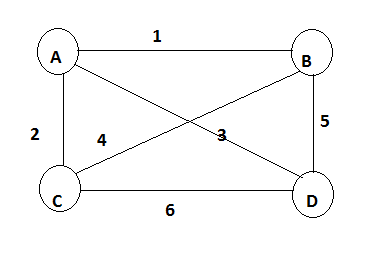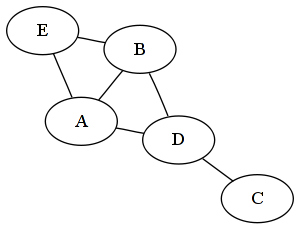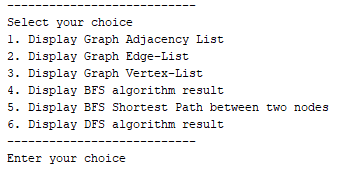# Python Learning Week 5

## ​​​​ Implementation of Breath First Search and Depth First Search

Background

Depth-First Search

The first algorithm I will be discussing is Depth-First search which as the name hints​​ at, explores possible vertices (from a supplied root) down each branch before backtracking. This property allows the algorithm to be implemented succinctly in both iterative and recursive forms. Below is a listing of the actions performed upon each visit to a node.

• Mark the current vertex as being visited.

• Explore each adjacent vertex that is not included in the visited set.

Connected Component

The implementation below uses the stack data-structure to build-up and return a set of vertices that are accessible within the subjects connected component. Using Python’s overloading of the subtraction operator to remove items from a set, we are able to add only the unvisited adjacent vertices.

defdfs(graph,start):

visited,stack=set(),[start]

whilestack:

vertex=stack.pop()

ifvertexnotinvisited:

stack.extend(graph[vertex]-visited)

returnvisited

dfs(graph,'A')# {'E', 'D', 'F', 'A', 'C', 'B'}

The second implementation provides the same functionality as the first, however, this time we are using the​​ more succinct recursive form. Due to a common Python gotcha with default parameter values being created only once, we are required to create a new visited set on each user invocation. Another Python language detail is that function variables are passed by reference, resulting in the visited mutable set not having to reassigned upon each recursive call.

defdfs(graph,start,visited=None):

ifvisitedisNone:

visited=set()

fornextingraph[start]-visited:

dfs(graph,next,visited)

returnvisited

dfs(graph,'C')# {'E', 'D', 'F', 'A', 'C', 'B'}

Paths

We are able to tweak both of the previous implementations to return all possible paths between a start and​​ goal vertex. The implementation below uses the stack data-structure again to iteratively solve the problem, yielding each possible path when we locate the goal. Using a generator allows the user to​​ only compute the desired amount of alternative paths.

defdfs_paths(graph,start,goal):

stack=[(start,[start])]

whilestack:

(vertex,path)=stack.pop()

fornextingraph[vertex]-set(path):

ifnext==goal:

yieldpath+[next]

else:

stack.append((next,path+[next]))

list(dfs_paths(graph,'A','F'))# [['A', 'C', 'F'], ['A', 'B', 'E', 'F']]

The implementation below uses the recursive approach calling the ‘yield from’ PEP380 addition to return the invoked​​ located paths. Unfortunately the version of Pygments installed on the server at this time does not include the updated keyword combination.

defdfs_paths(graph,start,goal,path=None):

ifpathisNone:

path=[start]

ifstart==goal:

yieldpath

fornextingraph[start]-set(path):

yieldfromdfs_paths(graph,next,goal,path+[next])

list(dfs_paths(graph,'C','F'))# [['C', 'F'], ['C', 'A', 'B', 'E', 'F']]

Breath-First Search

An alternative algorithm called Breath-First search provides us​​ with the ability to return the same results as DFS but with the added guarantee to return the shortest-path first. This algorithm is a little more tricky to implement in a recursive manner instead using the queue data-structure, as such I will only being documenting the iterative approach. The actions performed per each explored vertex are the same as the depth-first implementation, however, replacing the stack with a queue will instead explore the breadth of a vertex depth before moving on. This behavior​​ guarantees that the first path located is one of the shortest-paths present, based on number of edges being the cost factor.

Connected Component

Similar to the iterative DFS implementation the only alteration required is to remove the next item from the beginning of the list structure instead of the stacks last.

defbfs(graph,start):

visited,queue=set(),[start]

whilequeue:

vertex=queue.pop(0)

ifvertexnotinvisited:

queue.extend(graph[vertex]-visited)

returnvisited

bfs(graph,'A')# {'B',​​ 'C', 'A', 'F', 'D', 'E'}

Paths

This implementation can again be altered slightly to instead return all possible paths between two vertices, the first of which being one of the shortest such path.

defbfs_paths(graph,start,goal):

queue=[(start,[start])]

whilequeue:

(vertex,path)=queue.pop(0)

fornextingraph[vertex]-set(path):

ifnext==goal:

yieldpath+[next]

else:

queue.append((next,path+[next]))

list(bfs_paths(graph,'A','F'))# [['A', 'C', 'F'], ['A', 'B', 'E', 'F']]

Knowing that the shortest path will be​​ returned first from the BFS path generator method we can create a useful method which simply returns the shortest path found or ‘None’ if no path exists. As we are using a generator this in theory should provide similar performance results as just breaking​​ out and returning the first matching path in the BFS implementation.

defshortest_path(graph,start,goal):

try:

returnnext(bfs_paths(graph,start,goal))

exceptStopIteration:

returnNone

shortest_path(graph,'A','F')# ['A', 'C', 'F']

Define Class of Agent of​​ following graphclass Agent:

​​ ​​ ​​​​ A =[0,1,2,3,4]  ​​ ​​​​ B =[1,0,5,6,7]  ​​ ​​​​ C=[2,5,0,8,9]  ​​ ​​​​ D=[3,6,8,0,10]  ​​ ​​​​ E=[4,7,9,10,0]

​​ ​​ ​​​​ city = 0

#initializes at starting city

​​ ​​ ​​​​ cities = [A, B, C, D, E]

​​ ​​ ​​​​ total = 0

# Define constructor

​​ ​​ ​​​​ def __init__(self,​​ initCity):

​​ ​​ ​​ ​​ ​​ ​​ ​​​​ print "Distances from the initial city:", self.cities[initCity]

self.removeTravelled(initCity)

#Remove the travelled cities from list

​​ ​​ ​​​​ def removeTravelled(self, city):

print"Removing the indexed city"

self.current=self.cities[city]

​​​​  ​​ ​​ ​​ ​​ ​​​​ del self.A[city]

​​ ​​ ​​ ​​ ​​ ​​ ​​​​ del self.B[city]

​​ ​​ ​​ ​​ ​​ ​​ ​​​​ del self.C[city]

​​ ​​ ​​ ​​ ​​ ​​ ​​​​ del self.D[city]

​​ ​​ ​​ ​​ ​​ ​​ ​​​​ del self.E[city]

​​ ​​ ​​ ​​ ​​ ​​ ​​​​ del self.cities[city]

#find next index from listto be removed

​​ ​​ ​​​​ def findNext(self):

​​ ​​ ​​ ​​ ​​ ​​ ​​​​ index = 0  ​​ ​​ ​​ ​​ ​​ ​​​​ temp = 0

​​ ​​ ​​ ​​​​  ​​ ​​​​ min = self.current

​​ ​​ ​​ ​​ ​​ ​​ ​​​​ print self.current

​​ ​​ ​​ ​​ ​​ ​​ ​​​​ while temp<len(self.current):

​​ ​​ ​​ ​​ ​​ ​​ ​​ ​​ ​​ ​​ ​​​​ if min>self.current[temp] and self.current[temp]!=0:

​​ ​​ ​​ ​​ ​​ ​​ ​​ ​​ ​​ ​​ ​​ ​​ ​​ ​​ ​​​​ min = self.current[temp]

​​ ​​ ​​ ​​ ​​ ​​ ​​ ​​ ​​ ​​ ​​ ​​ ​​ ​​ ​​​​ index =temp

​​ ​​ ​​ ​​ ​​ ​​ ​​ ​​ ​​ ​​ ​​​​ temp= temp+1

print"The shortest distance is:", min

self.total=self.total+min

print"index is: ", index

​​ ​​ ​​ ​​ ​​ ​​ ​​​​ return index

​​ ​​ ​​ ​​ ​​ ​​ ​​​​ ###### Main ######

a = input("Please enter The node from where you want to start?")

agent= Agent(a) #making instant

for i in range (1,5):

​​ ​​ ​​​​ index = agent.findNext()

agent.removeTravelled(index)

else:

print"The total distance is : ", agent.total

Write a program in python to implement given Graph, BFS and DFS. ​​ Output must be as given belowImplement given graph in​​ python. Traverse Graph using DFS traversals. The starting node is ‘Frankfurt’ and goal node is ‘Munchen’.Check solution here Python Learning Week 5 (Solution)